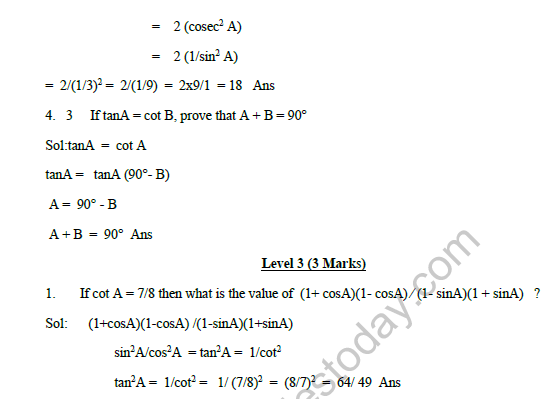# CBSE Class 10 Mathematics Trigonometry Assignment Set B

Read and download free pdf of CBSE Class 10 Mathematics Trigonometry Assignment Set B. Get printable school Assignments for Class 10 Mathematics. Standard 10 students should practise questions and answers given here for Chapter 8 Introduction To Trigonometry Mathematics in Grade 10 which will help them to strengthen their understanding of all important topics. Students should also download free pdf of Printable Worksheets for Class 10 Mathematics prepared as per the latest books and syllabus issued by NCERT, CBSE, KVS and do problems daily to score better marks in tests and examinations

## Assignment for Class 10 Mathematics Chapter 8 Introduction To Trigonometry

Class 10 Mathematics students should refer to the following printable assignment in Pdf for Chapter 8 Introduction To Trigonometry in standard 10. This test paper with questions and answers for Grade 10 Mathematics will be very useful for exams and help you to score good marks

### Chapter 8 Introduction To Trigonometry Class 10 Mathematics Assignment

CBSE Class 10 Mathematics Trigonometry Assignment Set B. Students are advised to refer to the attached assignments and practise them regularly. This will help them to identify their weak areas and will help them to score better in examination. Parents should download and give the assignments to their children for practice.

CASE STUDY

Two aeroplanes leave an airport, one after the other. After moving on runway, one flies due North and other flies due South. The speed of two aeroplanes are 400 km/hr and 500 km/hr respectively. Considering PQ as runway and A and B are any points in the path followed by two planesOn the basis of above answer the following questions

Question. Find tan𝜃, if ∠𝐴𝑃𝑄 = 𝜃
(a) 3/4
(b) 1/2
(c) 1/√2
(d) √3/2

Question. Find the value of cot𝐵
(a) 3/4
(b) 15/4
(c) 3/8
(b) 15/8

Question. Find the value of tan𝐴
(a) 3/4
(b) 4/3
(c) 1/√2
(d) √3/2

Question. Find the value of sec A
(a) 0
(b) 5/3
(c) 1/√2
(d) √3/2

Question. Find cosec B
(a) 17/8
(b) 8/17
(c) 12/5
(d) 5/12